Triangles, Theorems and Proofs: Tutoring Solution Chapter Exam

Exam Instructions:

Choose your answers to the questions and click 'Next' to see the next set of questions. You can skip questions if you would like and come back to them later with the yellow "Go To First Skipped Question" button. When you have completed the practice exam, a green submit button will appear. Click it to see your results. Good luck!

Page 1

Question 1 1. If triangle ABD is congruent to triangle CDB, CPCTC explains which of the following statements?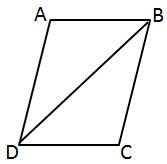Question 2 2. Which theorem can be used to establish congruence with the pictured triangles?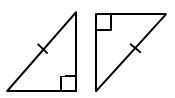Question 3 3. Which theorem or postulate can be used to establish congruence with the pictured triangles?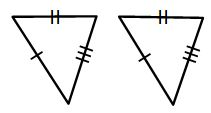Question 4 4. In the pictured triangle, XV is an angle bisector. What is the length of XY?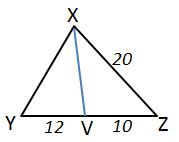Question 5 5. Which theorem or postulate can be used to establish congruence with the pictured triangles?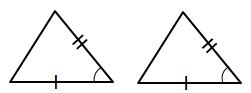Page 2

Question 6 6. If the pictured triangles are congruent, what reason can be given?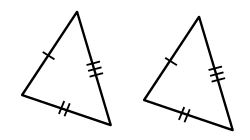Question 7 7. Triangle HYV and triangle AYB are similar by the AA similarity theorem. What is the value of x?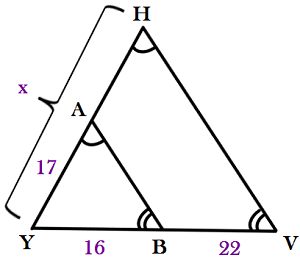Question 10 10. Find the measure of XZ: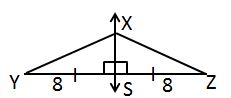Page 3

Question 11 11. What additional information do you need to prove that triangle ABC is congruent to triangle DEF using the HL theorem?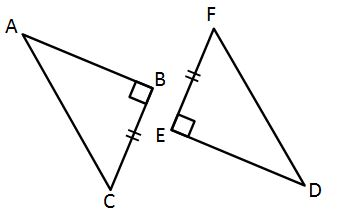Question 12 12. All of the following statements about the pictured triangle must be true, EXCEPT: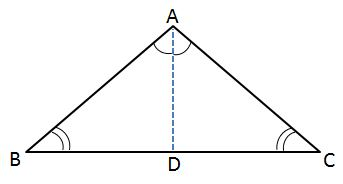Question 13 13. If triangle MNO is congruent to triangle PQR, CPCTC explains which of the following statements?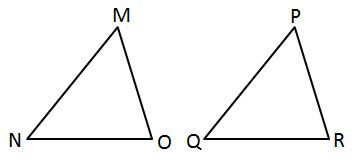Question 14 14. Find the measure of MN: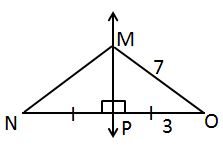Question 15 15. Which theorem can be used to establish congruence with the pictured triangles?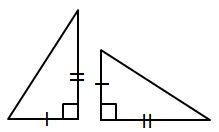Page 4

Question 17 17. What additional information do you need to be given to prove that triangle PQS is congruent to triangle SRP using the HA theorem?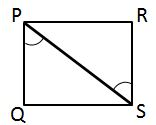Question 19 19. If triangle MNO is congruent to triangle STR, what must also be true?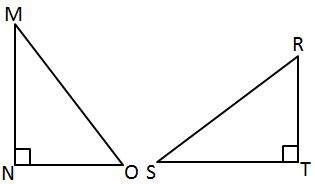Page 5

Question 21 21. If triangle ABC is congruent to triangle ADC, what must also be true?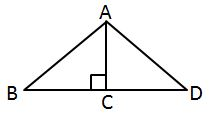Question 23 23. Triangle ABC is congruent to triangle XYZ. What reason can we use to explain why angle A is congruent to angle X?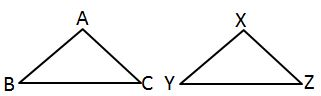Question 24 24. How can we explain that the pictured triangles are congruent?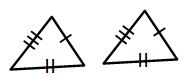Page 6

Question 26 26. Which theorem or postulate can be used to establish congruence with the pictured triangles?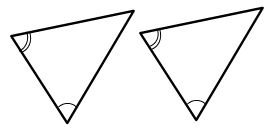Question 27 27. Study the given diagram. Find the value of x, the distance between T and E.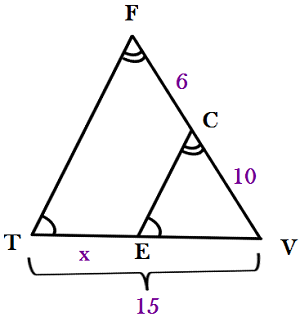Question 28 28. If triangle ABC is congruent to triangle RST, all of the following are true EXCEPT: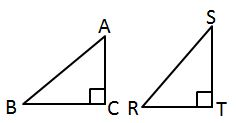Question 29 29. AD is a perpendicular bisector. Find the measure of AC: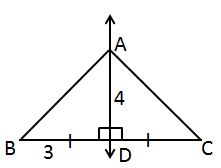Triangles, Theorems and Proofs: Tutoring Solution Chapter Exam Instructions

Choose your answers to the questions and click 'Next' to see the next set of questions. You can skip questions if you would like and come back to them later with the yellow "Go To First Skipped Question" button. When you have completed the practice exam, a green submit button will appear. Click it to see your results. Good luck!

Support